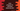# Java program to calculate electricity bill## Introduction :

In this tutorial, we will learn how to find the electricity bill using Java. I will show you different ways to solve this problem. Actually, the algorithm to solve that problem is the same but different ways to write the code.

### Program input and output :

This program will take the total number of electricity units as input and it will print the total cost for that unit.

We are considering the different cost for different units of electricity :

``````- For unit less than 100, it is 2\$
- For unit 101 to 200, it is 5\$
- For unit 201 to 300, it is 7\$
- For unit greater than 300, it is 9\$``````

### Formula to calculate the bill :

The program will take the total unit as the input. It will use the below formula to find out the cost :

``````if unit <= 100, cost = unit * 2
if 100 < unit <=200, cost = (100*2) + (unit - 100)*5
if 200 < unit <=300, cost = (100*2) + (100*5) + (unit - 200)*7
if unit > 300, cost = (100*2) + (100*5) + (100*7) + (unit - 300)*9``````

### Java program method 1:

We can simply convert the above conditions in if blocks and write our program :

``````class Main {
public static void main(String[] args) {
int unit = 400;
int cost = 0;

if (unit <= 100) cost = unit * 2;
if (100 < unit && unit <= 200) cost = (100 * 2) + (unit - 100) * 5;
if (200 < unit && unit <= 300) cost = (100 * 2) + (100 * 5) + (unit - 200) * 7;
if (unit > 300) cost = (100 * 2) + (100 * 5) + (100 * 7) + (unit - 300) * 9;

System.out.println("Total cost : "+cost);
}
}``````

It will print 2300 as the output.

### Method 2: Using if-else :

We can convert the above program to use if-else like below :

``````class Main {
public static void main(String[] args) {
int unit = 400;
int cost = 0;

if (unit <= 100) cost = unit * 2;
else if (100 < unit && unit <= 200) cost = (100 * 2) + (unit - 100) * 5;
else if (200 < unit && unit <= 300) cost = (100 * 2) + (100 * 5) + (unit - 200) * 7;
else if (unit > 300) cost = (100 * 2) + (100 * 5) + (100 * 7) + (unit - 300) * 9;

System.out.println("Total cost : "+cost);
}
}``````

### Method 3: Take unit as user input :

Using Scanner class, we can take the unit as user input :

``````import java.util.Scanner;

class Main {
public static void main(String[] args) {
Scanner sc = new Scanner(System.in);

System.out.println("Enter the total unit :");
int unit = sc.nextInt();

int cost = 0;

if (unit <= 100) cost = unit * 2;
else if (100 < unit && unit <= 200) cost = (100 * 2) + (unit - 100) * 5;
else if (200 < unit && unit <= 300) cost = (100 * 2) + (100 * 5) + (unit - 200) * 7;
else if (unit > 300) cost = (100 * 2) + (100 * 5) + (100 * 7) + (unit - 300) * 9;

System.out.println("Total cost : "+cost);
}
}``````

### Method 4: Using a different function :

The best way to solve these types of problems is by moving all calculation part to a new function :

``````import java.util.Scanner;

class Main {
static int getTotalCost(int unit) {
if (unit <= 100) return unit * 2;
else if (100 < unit && unit <= 200) return (100 * 2) + (unit - 100) * 5;
else if (200 < unit && unit <= 300) return (100 * 2) + (100 * 5) + (unit - 200) * 7;
else if (unit > 300) return (100 * 2) + (100 * 5) + (100 * 7) + (unit - 300) * 9;
else return 0;
}

public static void main(String[] args) {
Scanner sc = new Scanner(System.in);

System.out.println("Enter the total unit :");
int unit = sc.nextInt();

System.out.println("Total cost : " + getTotalCost(unit));
}
}``````

getTotalCost is a different function that takes unit as the input and returns the cost.

### Method 5: Using a different class :

This is the recommended way to solve problems like this. Create one utility class and move all utility functions to this :

``````class Util {
static int getTotalCost(int unit) {
if (unit <= 100) return unit * 2;
else if (100 < unit && unit <= 200) return (100 * 2) + (unit - 100) * 5;
else if (200 < unit && unit <= 300) return (100 * 2) + (100 * 5) + (unit - 200) * 7;
else if (unit > 300) return (100 * 2) + (100 * 5) + (100 * 7) + (unit - 300) * 9;
else return 0;
}
}``````

You can call this function from Main class as like below :

``````import java.util.Scanner;

class Main {
public static void main(String[] args) {
Scanner sc = new Scanner(System.in);

System.out.println("Enter the total unit :");
int unit = sc.nextInt();

System.out.println("Total cost : " + Util.getTotalCost(unit));
}
}``````